# Polka Dot Carpet Fractal

### Fractal Carpet

The Polka Dot Carpet Fractal was made by removing only one block from level one, which was coordinate (2,2). I only took out one block because I wanted to see how a simple design become more complicated going up each level.

Below is the equation used to calculate surface area of level 1, 2 and 3 of the Polka Dot Carpet Fractal.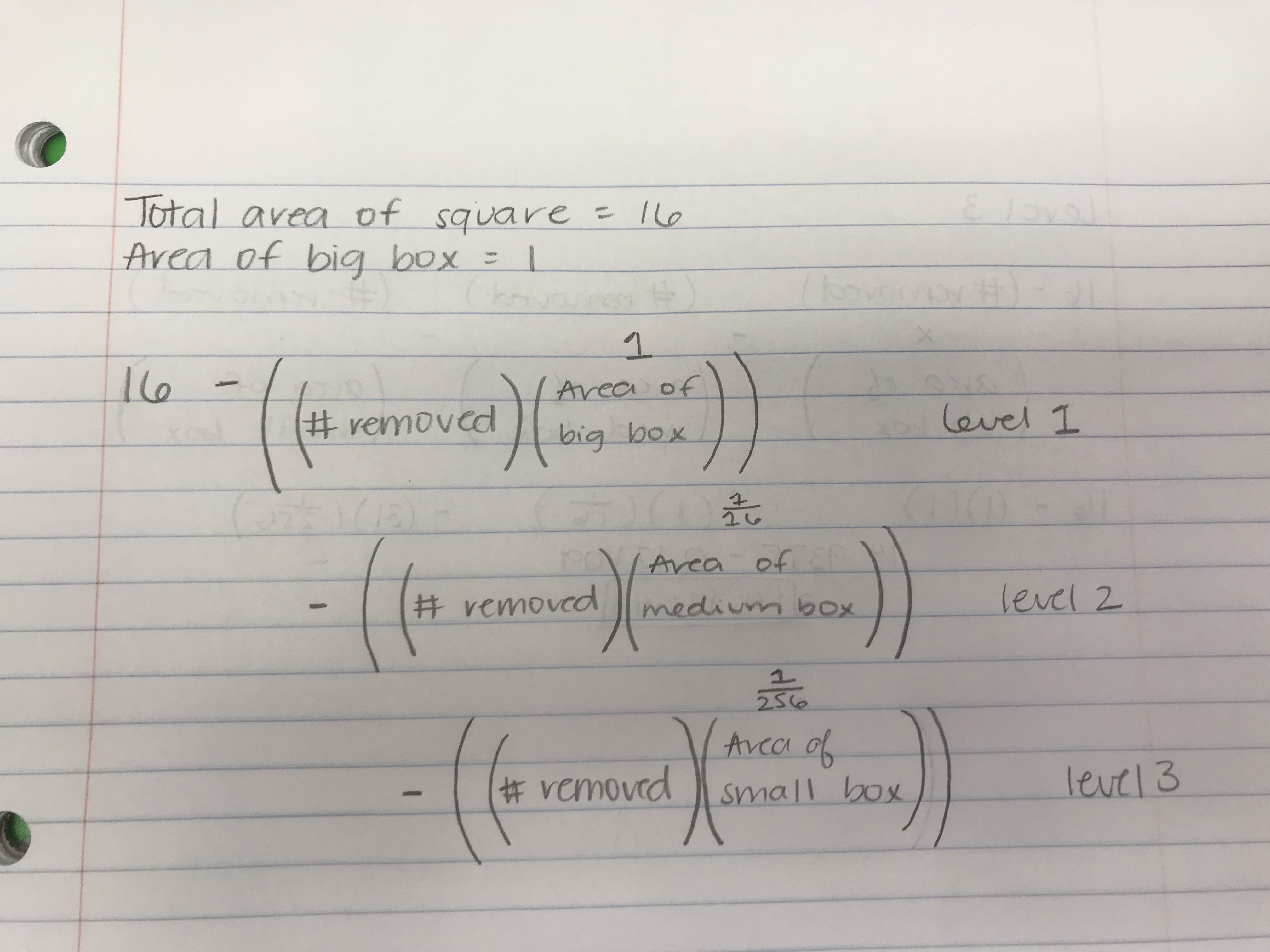Below are pictures of my level 1, 2 and 3 prints.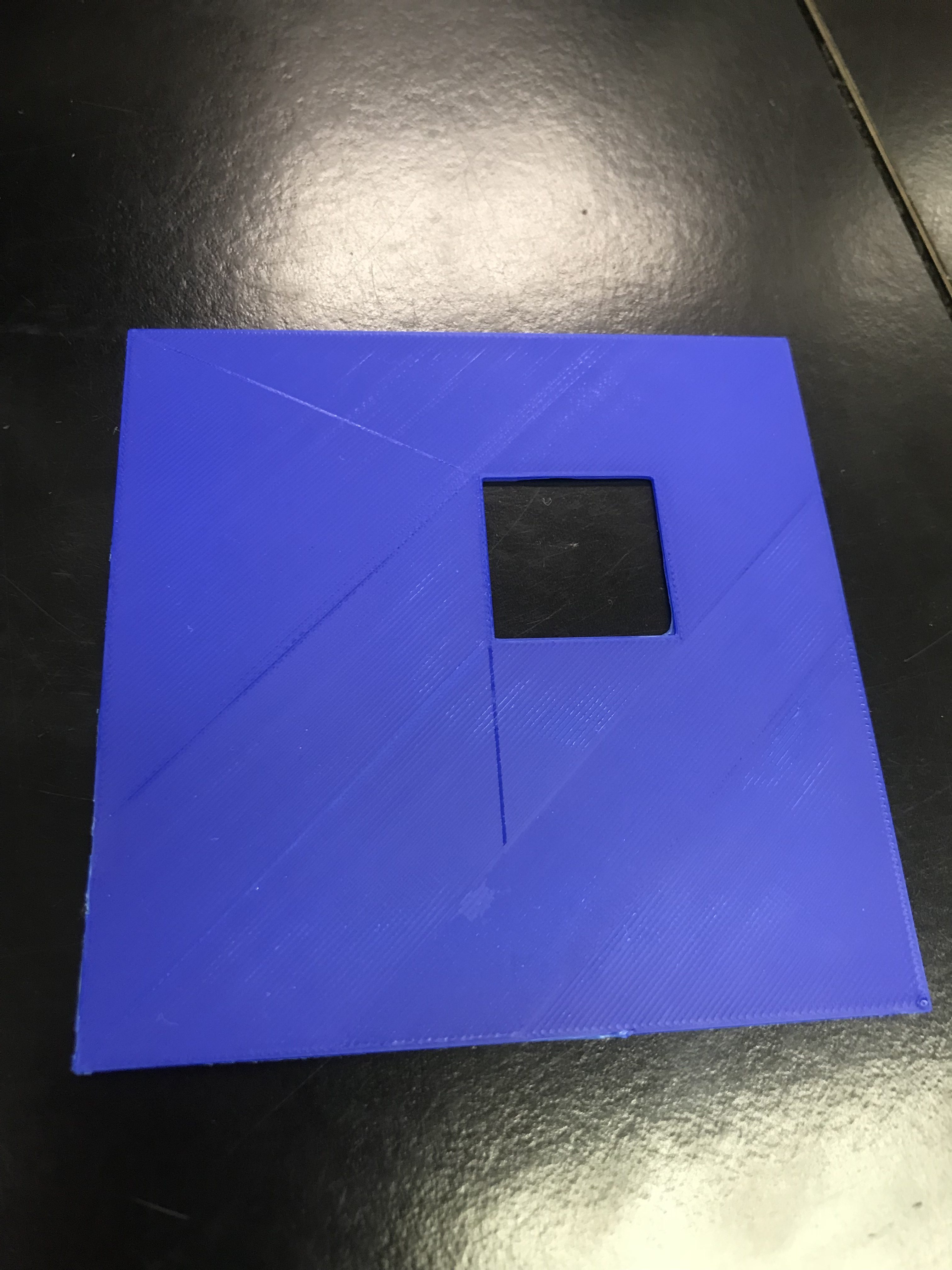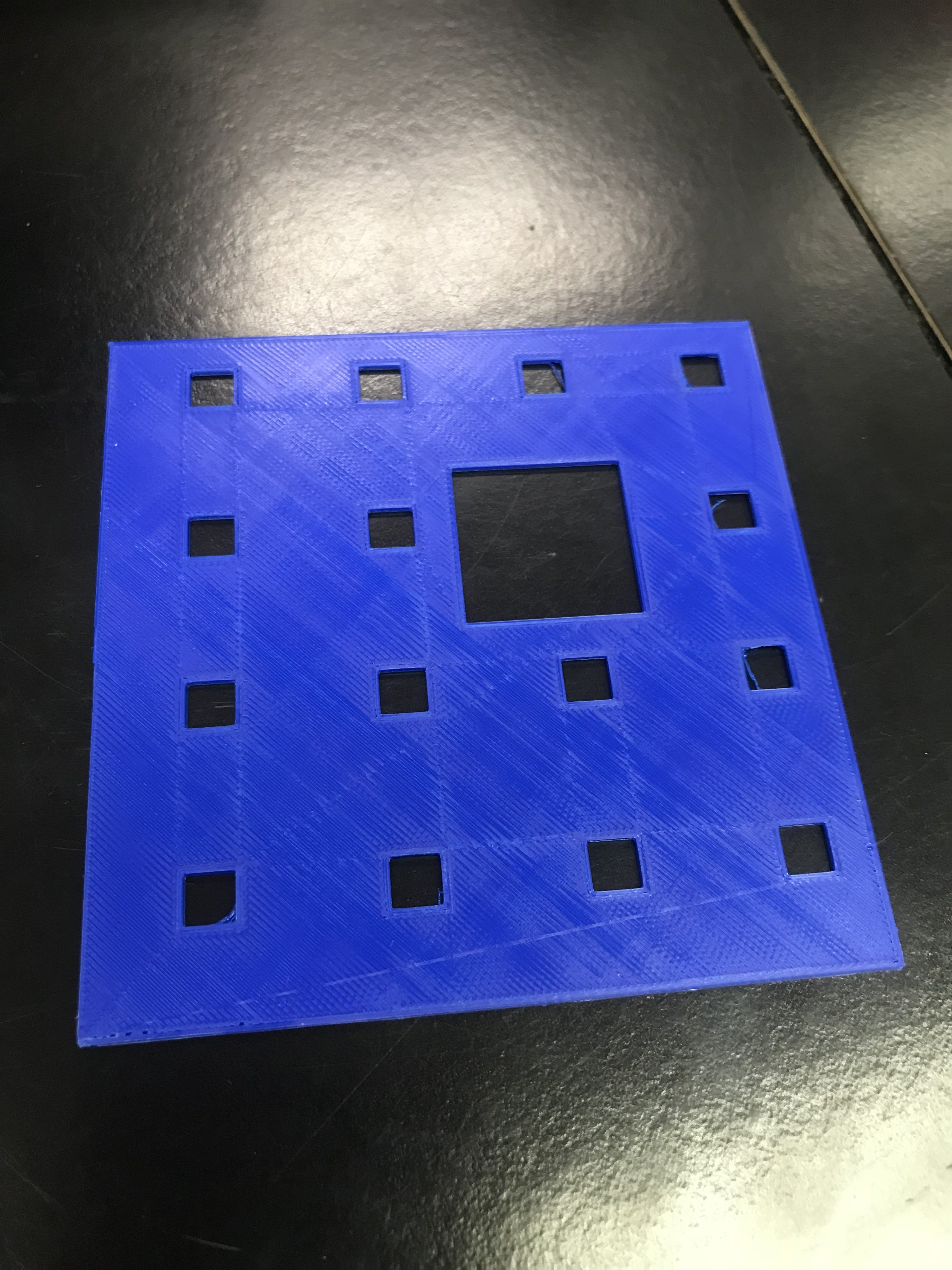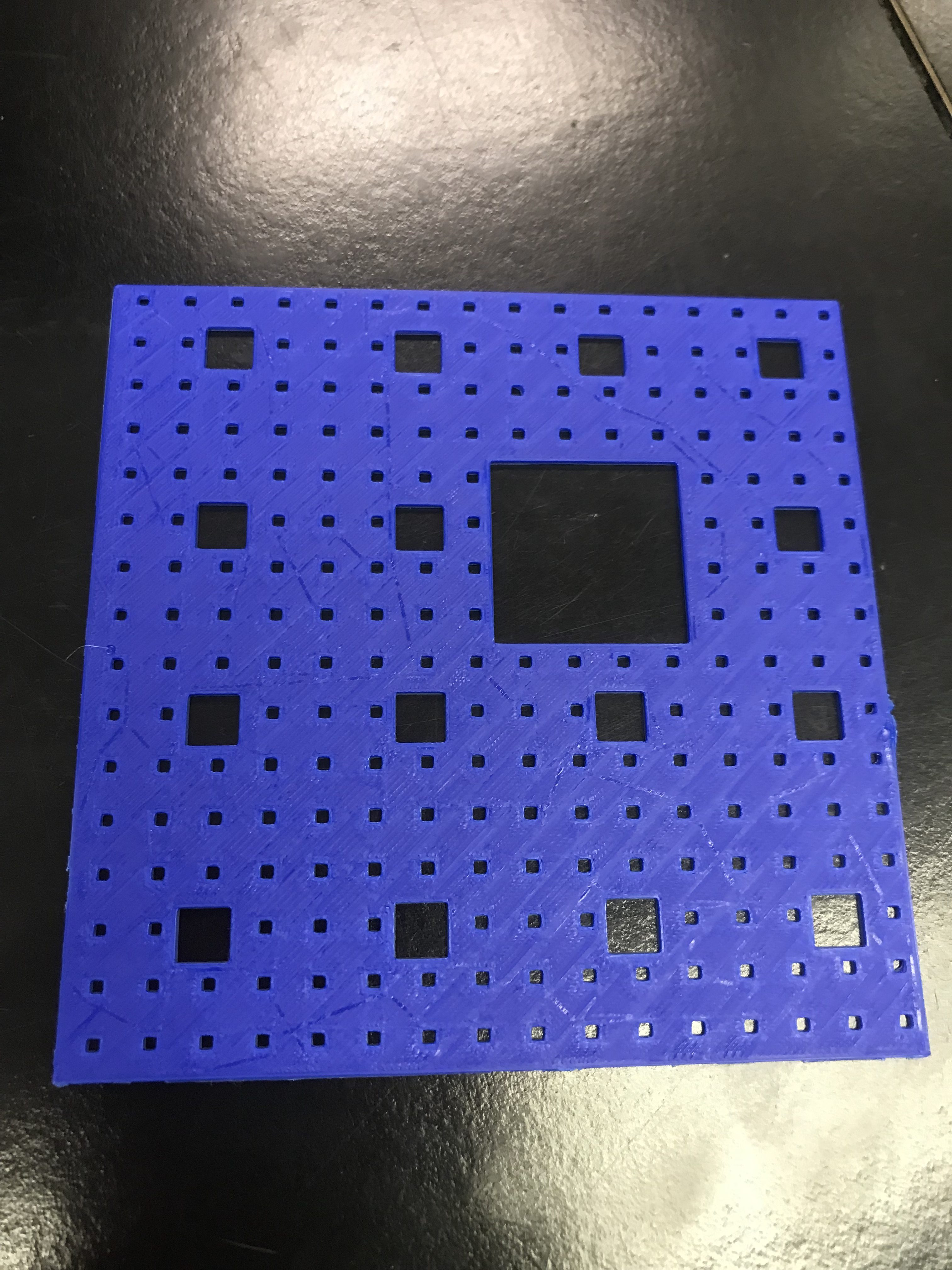### Surface Area Calculations

Below is my surface area calculations for levels 1, 2 and 3 using the formal that was first pictured.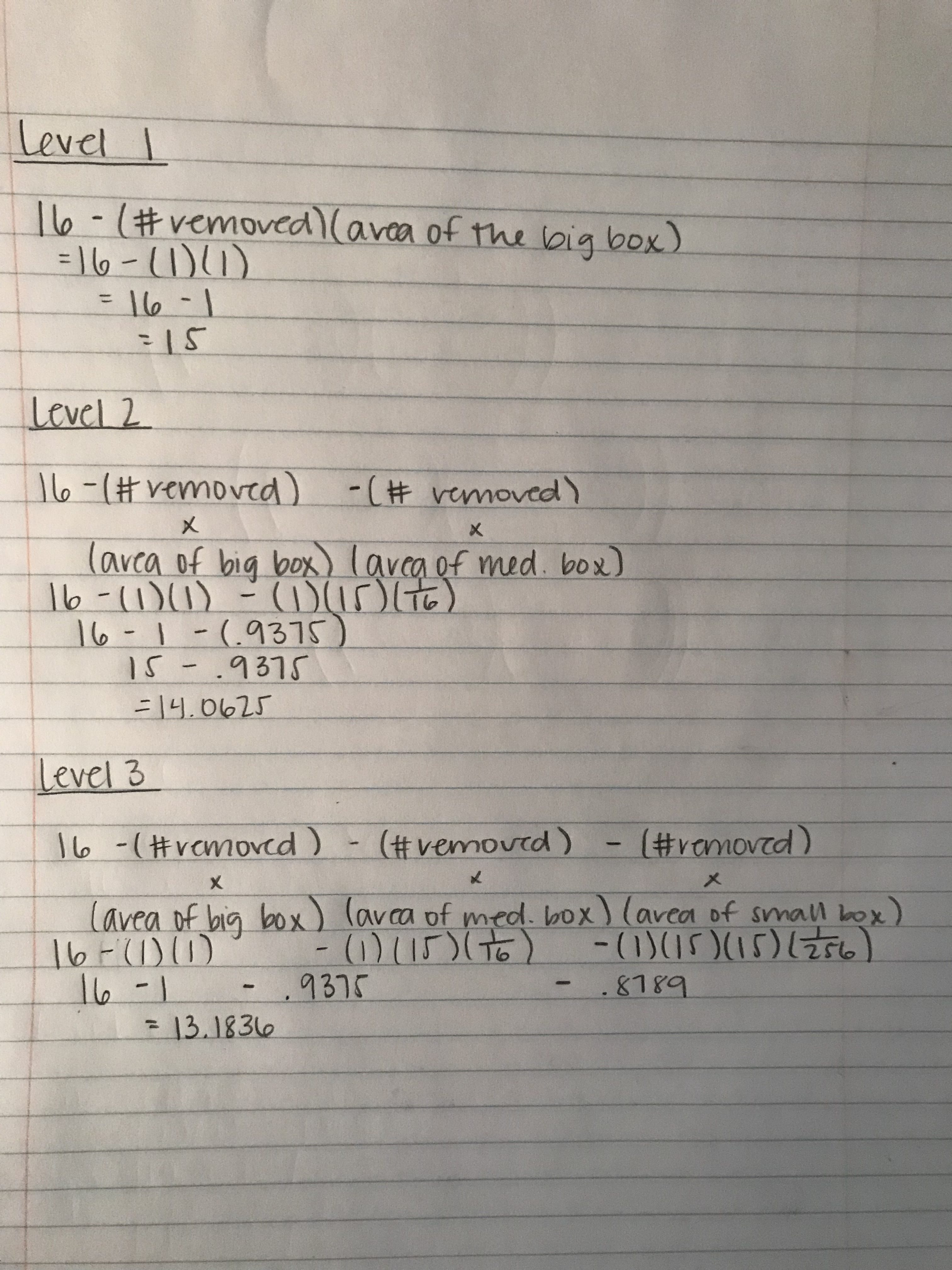### Sum of Infinite Series

Below is the sum of an infinite series. To calculate this, I had to find that a=1 and r =15/16 and then follow the equation of a + ar + ar^2 + ar^3 + ar^4… if |r| < 1, and then I had to plug in ‘a’ and ‘r’ in the formula of a x 1/(1- r) which ended up equally 16 so that cancelled out the initial area of 16, which equals to zero.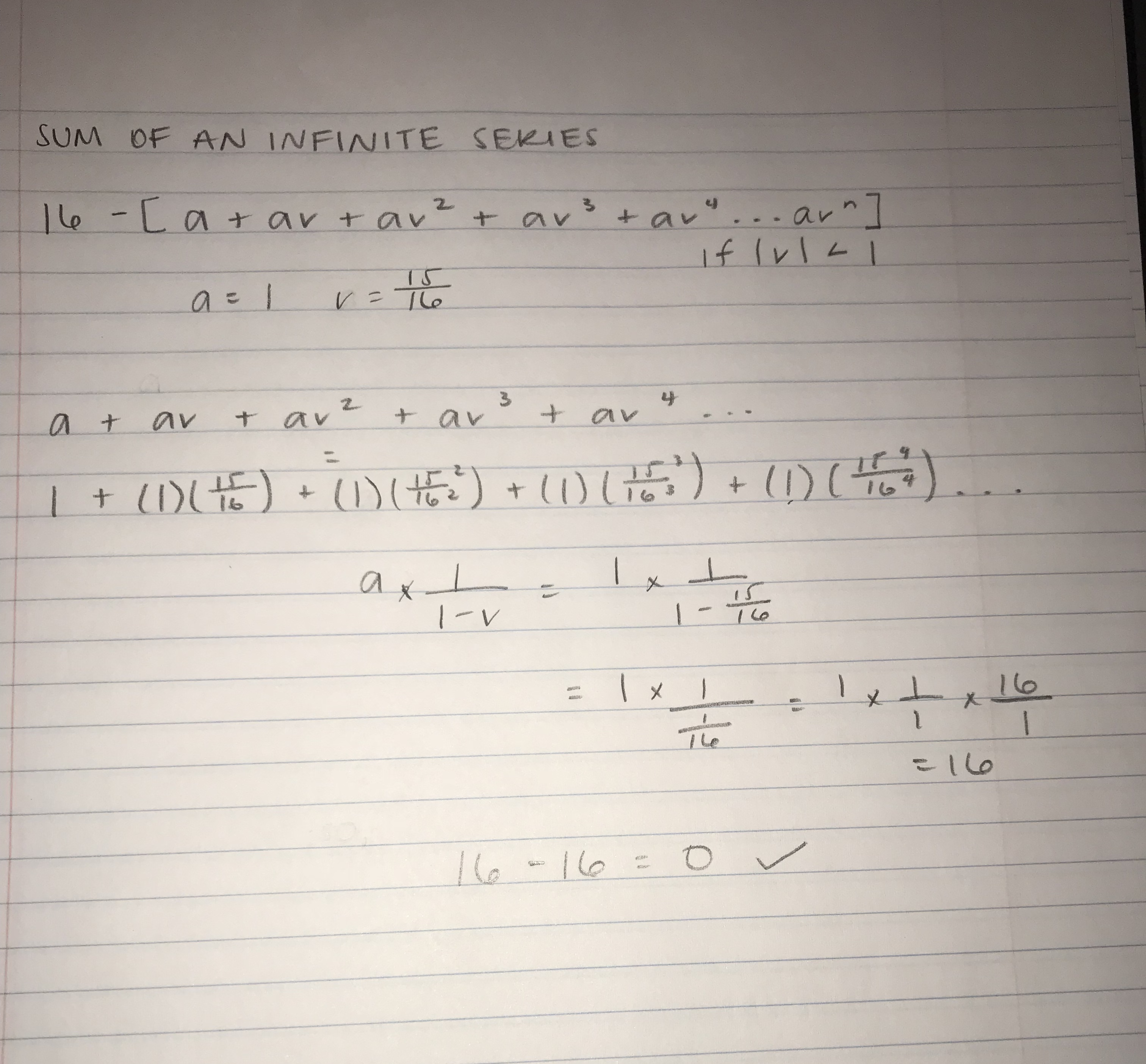### Dimension

The formula to find dimension is (scaled down factor)^(dimension)= 1/(number of copies) so S^D = 1/N.
In this case, the fractal is scaled down by 1/4. There are 15 copies within the whole fractal so the top left square drawn is 1/15 of the whole fractal. S = 1/4 and N = 15, which makes the equation look like (1/4)^D= 1/15. This also can equate to 4^D= 15 or log_4 (15)= D, which equals to 1.953.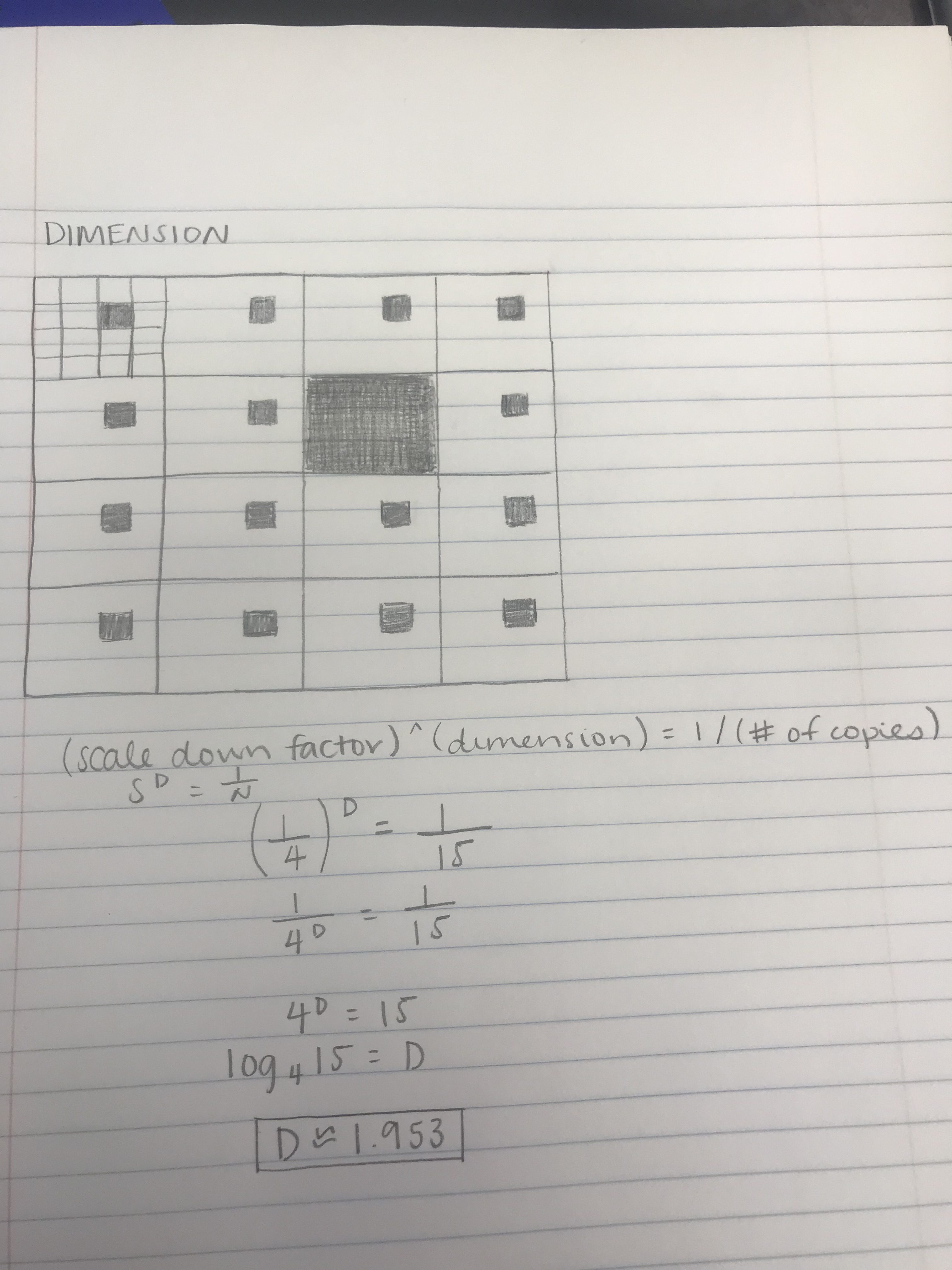You can view the Polka Dot Carpet Fractal on Thingiverse.